# Recursion in Java recursion Recursion is the process

• Slides: 62Recursion in Java recursion: Recursion is the process of defining something in terms of itself. Leonardo da Vinci 1452 – 1519 La Giaconda (Mona Lisa) Louvre, Paris. 1Why learn recursion ? • "cultural experience" - A different way of thinking on problems. • Can solve some kinds of problems better than iteration (loops). • Leads to elegant, simplistic, short Java code (when used well). • Many programming languages ("functional" languages such as Scheme, ML, and Haskell) use recursion exclusively (no loops). 2Why learn recursion ? Recursion is a key component of the our course assignments number 2 3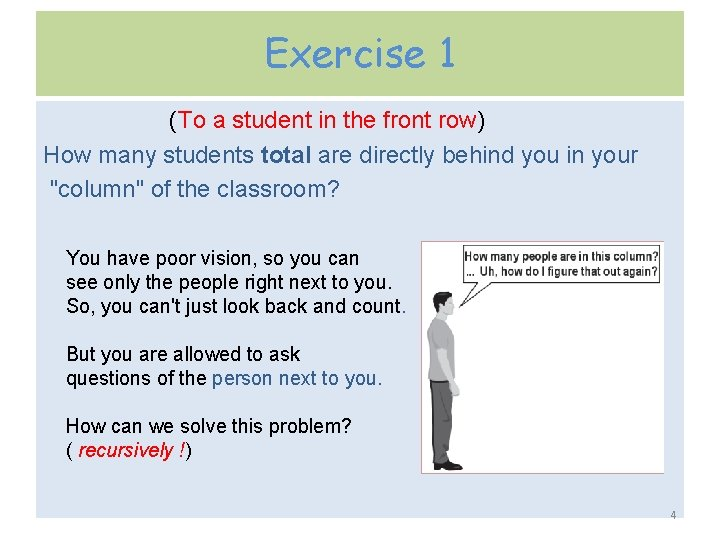Exercise 1 (To a student in the front row) How many students total are directly behind you in your "column" of the classroom? You have poor vision, so you can see only the people right next to you. So, you can't just look back and count. But you are allowed to ask questions of the person next to you. How can we solve this problem? ( recursively !) 4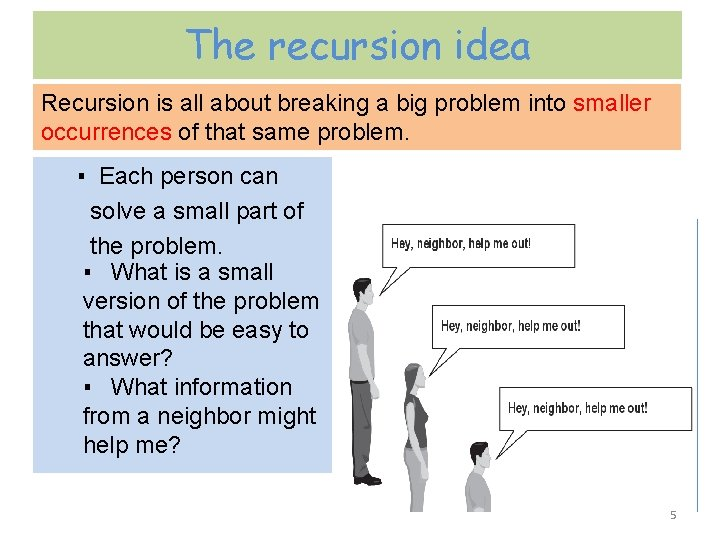The recursion idea Recursion is all about breaking a big problem into smaller occurrences of that same problem. ▪ Each person can solve a small part of the problem. ▪ What is a small version of the problem that would be easy to answer? ▪ What information from a neighbor might help me? 5Recursive algorithm • Number of people behind me: – If there is someone behind me, ask him/her how many people are behind him/her. - When they respond with a value N, then I will answer N + 1. – If there is nobody behind me, I will answer 0. 6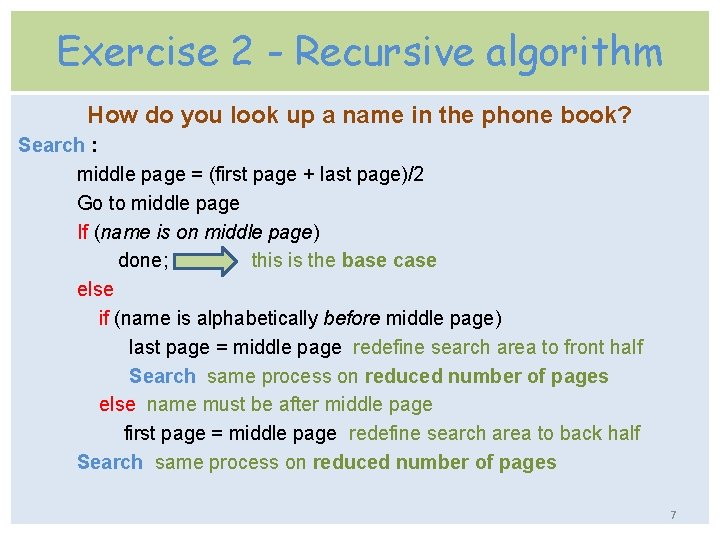Exercise 2 - Recursive algorithm How do you look up a name in the phone book? Search : middle page = (first page + last page)/2 Go to middle page If (name is on middle page) done; this is the base case else if (name is alphabetically before middle page) last page = middle page redefine search area to front half Search same process on reduced number of pages else name must be after middle page first page = middle page redefine search area to back half Search same process on reduced number of pages 7Recursive algorithm - Overview • Natural approach to some (not all) problems. • A recursive algorithm uses itself to solve one or more smaller identical problems. • Each successive call to itself must be a “ smaller version of itself ”. • A recursive algorithm must eventually terminate. A recursive algorithm must have at least one base, or stopping, case. A base case does not execute a recursive call. 8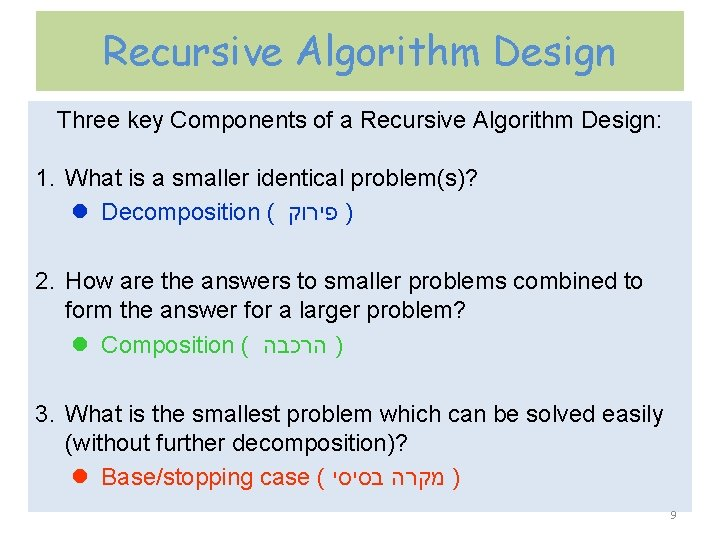Recursive Algorithm Design Three key Components of a Recursive Algorithm Design: 1. What is a smaller identical problem(s)? l Decomposition ( פירוק ) 2. How are the answers to smaller problems combined to form the answer for a larger problem? l Composition ( הרכבה ) 3. What is the smallest problem which can be solved easily (without further decomposition)? l Base/stopping case ( בסיסי מקרה ) 9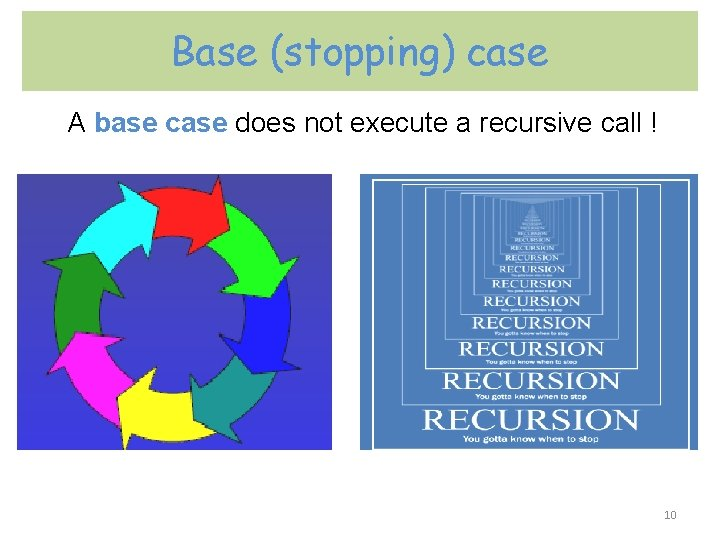Base (stopping) case A base case does not execute a recursive call ! 10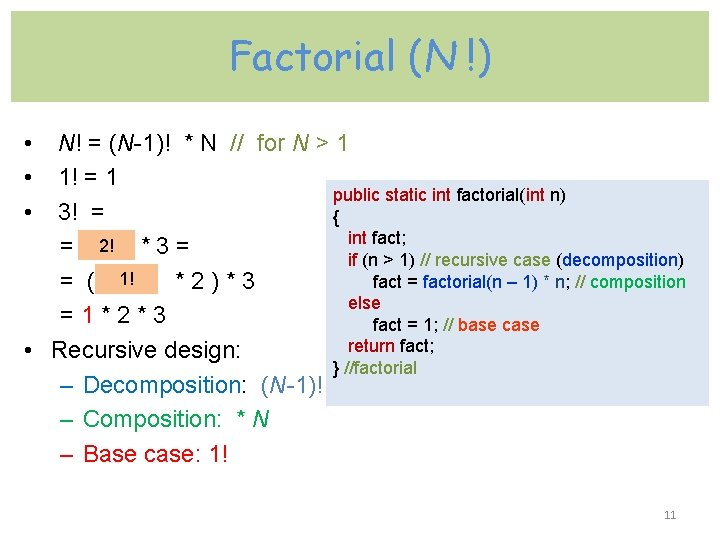Factorial (N !) • N! = (N-1)! * N // for N > 1 • 1! = 1 public static int factorial(int n) • 3! = { int fact; 2! = * 3 = if (n > 1) // recursive case (decomposition) 1! fact = factorial(n – 1) * n; // composition = ( * 2 ) * 3 else = 1 * 2 * 3 fact = 1; // base case return fact; • Recursive design: } //factorial – Decomposition: (N-1)! – Composition: * N – Base case: 1! 11public static int factorial(int 3) { int fact; if (n > 1) fact = factorial(2) * 3; else fact = 1; return fact; } Each recursive call to itself must be a “ smaller version of itself ”. public static int factorial(int 2) { int fact; if (n > 1) fact = factorial(1) * 2; else fact = 1; return fact; } A base case does not execute a recursive call and stops the recursion. public static int factorial(int 1) { int fact; if (n > 1) fact = factorial(n - 1) * n; else fact = 1; return fact; } 12public static int factorial(int 3) { int fact; if (n > 1) fact = factorial(2) * 3; else fact = 1; return fact; } return 2 return 6 public static int factorial(int 2) { int fact; if (n > 1) fact = factorial(1) * 2; else fact = 1; return fact; } return 1 public static int factorial(int 1) { int fact; if (n > 1) fact = factorial(n - 1) * n; else fact = 1; return fact; } 13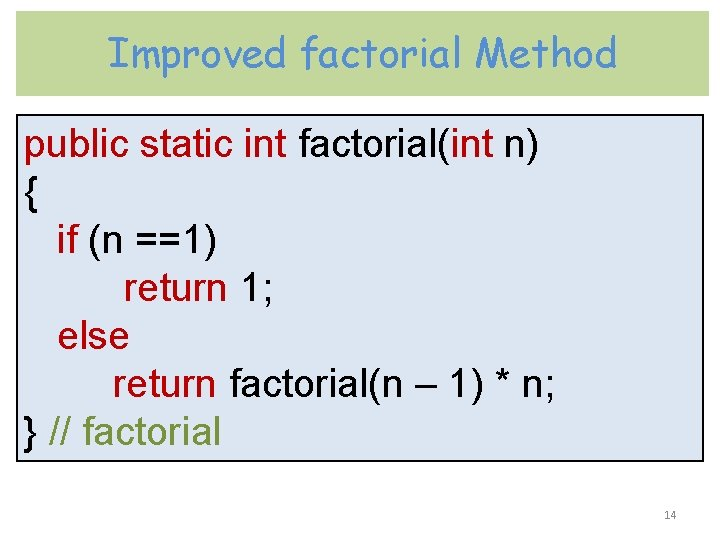Improved factorial Method public static int factorial(int n) { if (n ==1) return 1; else return factorial(n – 1) * n; } // factorial 14STACK ( מחסנית ) • A stack is a special area of memory where access can be made only from one end. You can only access and work with the top of the stack. • This is called a Last In First Out (LIFO) structure. • A stack has two fundamental operations - Push and Pop. The Push operation stores something on the top of the stack and the Pop operation retrieves something from the top of the stack. • Calling a method often means putting the parameter values on to the stack, returning from a method is essentially the reverse process. Top of the stack 15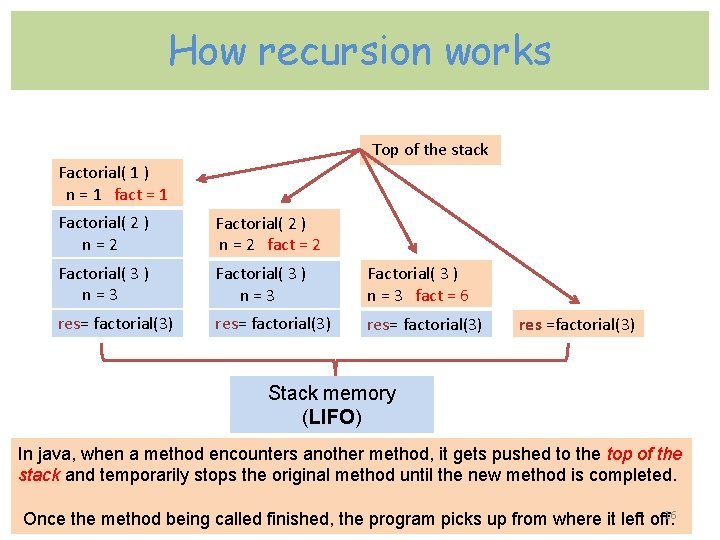How recursion works Top of the stack Factorial( 1 ) n = 1 fact = 1 Factorial( 2 ) n = 2 Factorial( 2 ) n = 2 fact = 2 Factorial( 3 ) n = 3 Factorial( 3 ) n = 3 fact = 6 res= factorial(3) res =factorial(3) Stack memory (LIFO) In java, when a method encounters another method, it gets pushed to the top of the stack and temporarily stops the original method until the new method is completed. 16 Once the method being called finished, the program picks up from where it left off.Arithmetic Series Calculation arithmetic series (sigma) recursive Sum : public static int sigma(int n) { if(n <= 1) return n; else return n + sigma(n-1); } // sigma Recursive design: Decomposition: (n-1) Composition: n + sigma(n-1) Base case: n=1 17If we call the sigma method with the Java statement : int sum = sigma(5) ; the else portion of the first method’s calls the method sigma again return 5 + sigma(4); push pop 18Calculation power y X = x*x*…*x y times Recursive definitions (assume non-negative y): public static int power(int x, int y) { if (y == 0) return 1; else return x*power(x, y– 1); } // power Xy = X*(Xy-1) Base case: x 0=1 19Calculation product of two non - negative integers without multiplication operator * ( we can use only ‘+’ and ‘-’ operators ) The product a*b is actually a +…+ a (b times). x * y = x + x + …+ x y times Recursive definitions : x * y = y + ( x – 1) * y Base case: 0 * Y = 0 public static int rec. Mult( int x, int y ) { if( x == 0) return 0; return y + rec. Mult( x-1, y); } // rec. Mult 20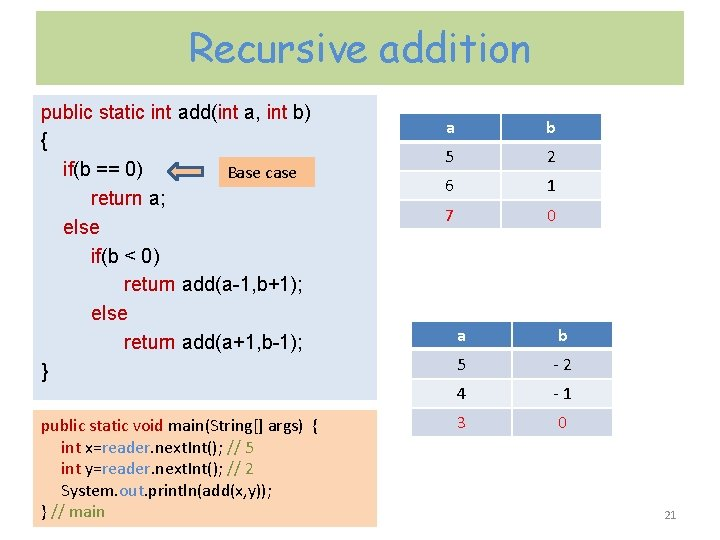Recursive addition public static int add(int a, int b) { if(b == 0) Base case return a; else if(b < 0) return add(a-1, b+1); else return add(a+1, b-1); } public static void main(String[] args) { int x=reader. next. Int(); // 5 int y=reader. next. Int(); // 2 System. out. println(add(x, y)); } // main a b 5 2 6 1 7 0 a b 5 - 2 4 - 1 3 0 21Recursive subtraction public static int sub(int a, int b) { if(b == 0) Base case return a; else if(b < 0) return sub(a+1, b+1); else return sub(a-1, b-1); } public static void main(String[] args) { int x=reader. next. Int(); // 5 int y=reader. next. Int(); // 2 System. out. println(sub(x, y)); } //main a b 5 2 4 1 3 0 a b 5 - 2 6 - 1 7 0 22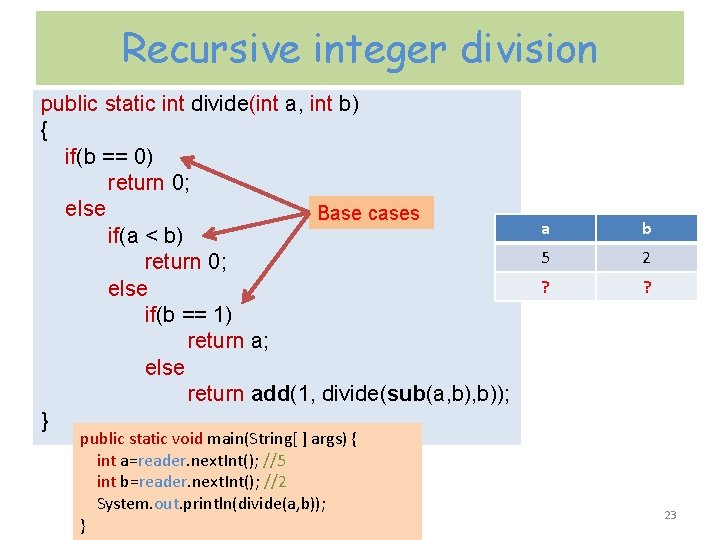Recursive integer division public static int divide(int a, int b) { if(b == 0) return 0; else Base cases if(a < b) return 0; else if(b == 1) return a; else return add(1, divide(sub(a, b)); } public static void main(String[ ] args) { int a=reader. next. Int(); //5 int b=reader. next. Int(); //2 System. out. println(divide(a, b)); } a b 5 2 ? ? 23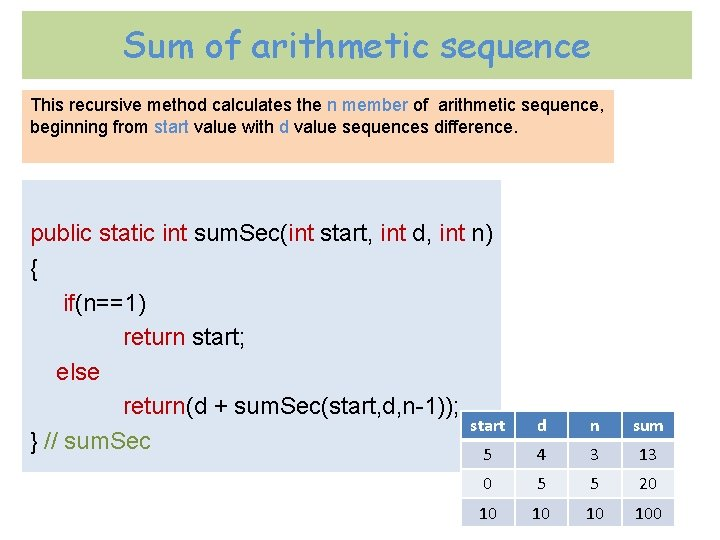Sum of arithmetic sequence This recursive method calculates the n member of arithmetic sequence, beginning from start value with d value sequences difference. public static int sum. Sec(int start, int d, int n) { if(n==1) return start; else return(d + sum. Sec(start, d, n-1)); start } // sum. Sec 5 d n sum 4 3 13 0 5 5 20 10 100 24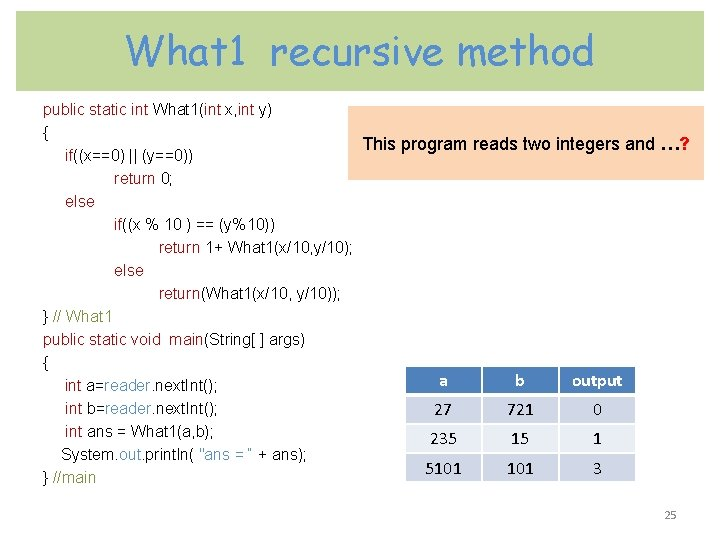What 1 recursive method public static int What 1(int x, int y) { This program reads two integers and …? if((x==0) || (y==0)) return 0; else if((x % 10 ) == (y%10)) return 1+ What 1(x/10, y/10); else return(What 1(x/10, y/10)); } // What 1 public static void main(String[ ] args) { a b output int a=reader. next. Int(); int b=reader. next. Int(); 27 721 0 int ans = What 1(a, b); 235 15 1 System. out. println( "ans = “ + ans); 5101 3 } //main 25Fibonacci series • Fibonacci was born on 1170 in Pisa, Italy and died on 1250. His real name is Leonardo Pisano. • The N - th Fibonacci number is the sum of the previous two Fibonacci numbers : 0 1 2 3 4 5 6 7 0, 1, 1, 2, 3, 5, 8, 13, … Number element in series • Recursive Design: – Decomposition & Composition • fibonacci(n) = fibonacci(n-1) + fibonacci(n-2) – Base case: public static fibonacci(int n) • fibonacci(0) = 0 { • fibonacci(1) = 1 if (n<=1) Binary Recursion is a process where function is called twice at a time instead of once at a time. return n; return fibonacci(n-1) + fibonacci(n-2); } // fibonacci 26Fibonacci series n=5 5 3 2 1 1 1 0 0 Binary recursion( כפולה )רקורסיה 27Recursion and loops public static int rec. Loop(int num) public static void main(String[ ] args) { { if(num == 1) System. out. println("enter an integer -> "); int x=reader. next. Int(); return 1; System. out. println("what= “ + rec. Loop(x)); int sum = num; } // main for(int i=1; i<num; i++) sum = sum + rec. Loop(i); return sum; input output 1 1 } // rec. Loop 2 3 3 7 4 15 28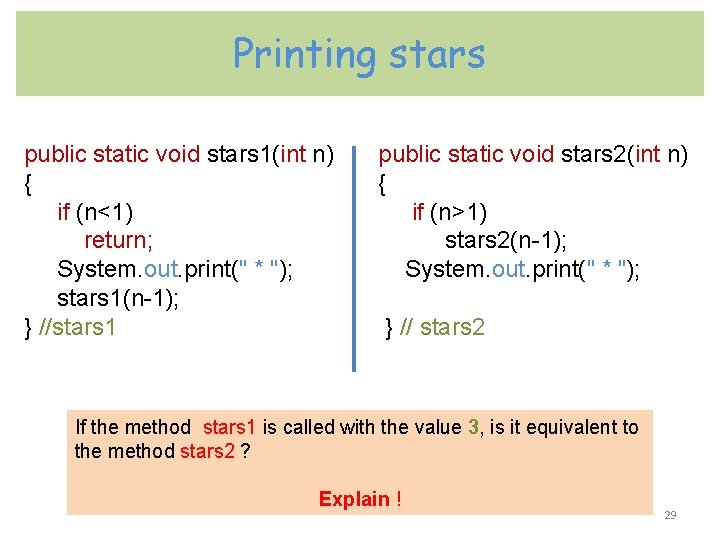Printing stars public static void stars 1(int n) { if (n<1) return; System. out. print(" * "); stars 1(n-1); } //stars 1 public static void stars 2(int n) { if (n>1) stars 2(n-1); System. out. print(" * "); } // stars 2 If the method stars 1 is called with the value 3, is it equivalent to the method stars 2 ? Explain ! 29Invoke stars 1 static void stars 1( 3 ) { if (n<1) FALSE return; System. out. print(" * "); stars 1(2); } First output : * static void stars 1( 2 ) { if (n<1) FALSE return; System. out. print(" * "); stars 1(1); } Second output : ** static void stars 1( 1 ) { if (n<1) FALSE return; System. out. print(" * "); stars 1(0); } Third output : *** static void stars 1( 0 ) { if (n<1) TRUE return; System. out. print(" * "); stars 1(n-1); } 30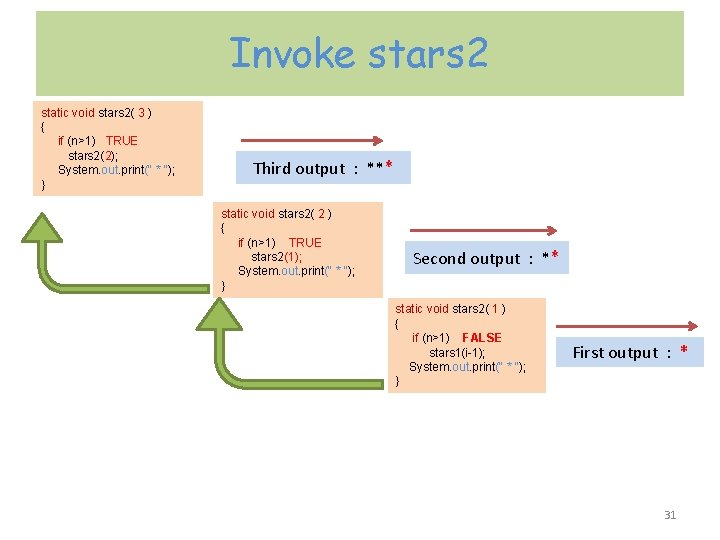Invoke stars 2 static void stars 2( 3 ) { if (n>1) TRUE stars 2(2); System. out. print(" * "); } Third output : *** static void stars 2( 2 ) { if (n>1) TRUE stars 2(1); System. out. print(" * "); } Second output : ** static void stars 2( 1 ) { if (n>1) FALSE stars 1(i-1); System. out. print(" * "); } First output : * 31Mutual recursion • Mutual Recursion ( ) רקורסיה הדדית is the kind of recursion where recursive methods calling each other. For example: YES method calling NO method and NO method calling YES method recursively. public static void YES (int num) { System. out. println(“yes”); if (num>1) NO(num-1); } //YES public static void NO (int num) { System. out. println(“no”); if (num>1) YES(num-1); } // NO yes no invoke YES(5) would yes produce next output: no yes 32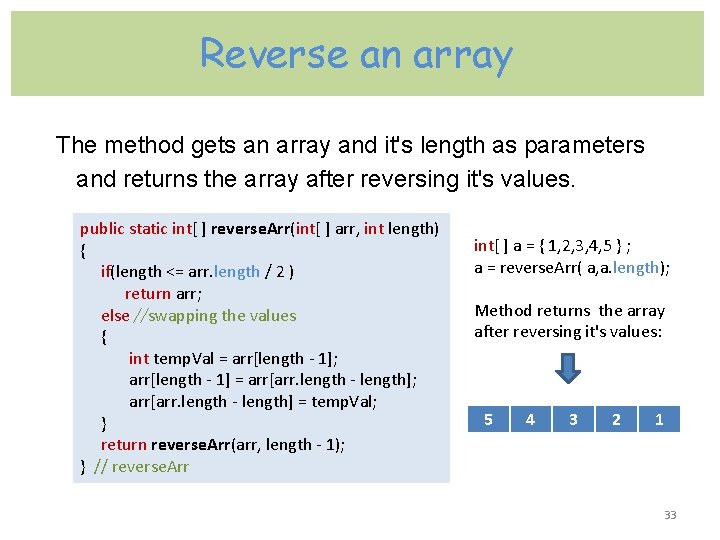Reverse an array The method gets an array and it's length as parameters and returns the array after reversing it's values. public static int[ ] reverse. Arr(int[ ] arr, int length) int[ ] a = { 1, 2, 3, 4, 5 } ; { a = reverse. Arr( a, a. length); if(length <= arr. length / 2 ) return arr; Method returns the array else //swapping the values after reversing it's values: { int temp. Val = arr[length - 1]; arr[length - 1] = arr[arr. length - length]; arr[arr. length - length] = temp. Val; 5 4 3 2 1 } return reverse. Arr(arr, length - 1); } // reverse. Arr 33Reverse a string public static String reverse. String(String s) { if (s. length() <= 1) return s; return reverse. String (s. substring(1)) + s. char. At(0); } // reverse. String a b c d + d System. out. print("Enter the string: "); String str = reader. next(); // abcd System. out. print("The reverse string is" + reverse. String(str)); would produce next output: dcba 34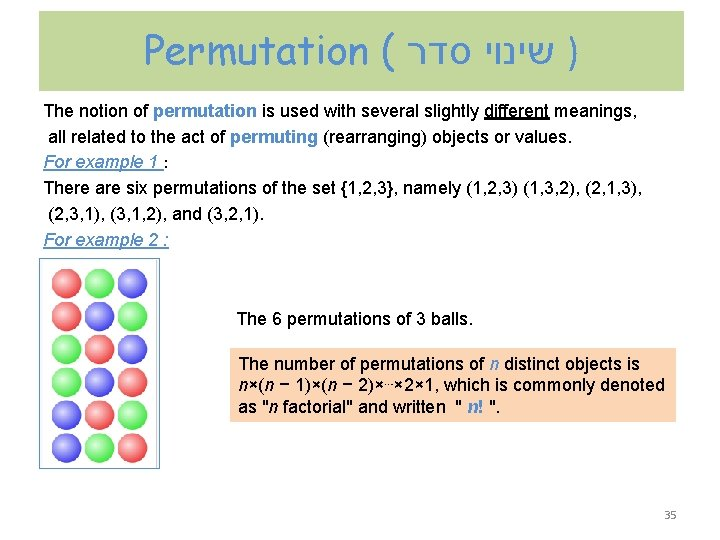Permutation ( ) שינוי סדר The notion of permutation is used with several slightly different meanings, all related to the act of permuting (rearranging) objects or values. For example 1 : There are six permutations of the set {1, 2, 3}, namely (1, 2, 3) (1, 3, 2), (2, 1, 3), (2, 3, 1), (3, 1, 2), and (3, 2, 1). For example 2 : The 6 permutations of 3 balls. The number of permutations of n distinct objects is n×(n − 1)×(n − 2)×⋯× 2× 1, which is commonly denoted as "n factorial" and written " n! ". 35Anagram An anagram( אנגרמה ) of a word is a permutation of its letters : the result of rearranging the letters of a word to produce a new word, using all the original letters exactly once. The original word is known as the subject of the anagram. For example : If the subject word is “abc” , then all anagrams of a word is the next set: abc acb bac bca cab cba 3! = 1 x 2 x 3 = 6 36Anagram and recursion We can use recursion to generate all the anagrams of a word of any length. The algorithm works as follows: put every character in the string as first letter, and recursively find all anagrams of the remaining letters. Given abc, we would place a in front of all two permutations of bc - bc and cb to arrive at abc and acb. Then we would place b in front of all two permutations of ac – ac and ca to arrive bac and bca and finally c in front of two permutations of ab – ab and ba. abc acb bac bca cab cba Thus, there will be three recursive calls to display all permutations of a three-letter word. The base case of our recursion would be when we reach a word with just one letter. 37Anagram solution public static void print. Anagrams(String prefix, String word) { if(word. length() <= 1) System. out. println(prefix + word); else { for(int i = 0; i < word. length(); i++) { String cur = word. substring(i, i + 1); String before = word. substring(0, i); // letters before cur String after = word. substring(i + 1); // letters after cur print. Anagrams(prefix + cur, before + after); } // for } // else } // print. Anagram public static void main(String[ ] args) { print. Anagrams("", "abc"); } // main 38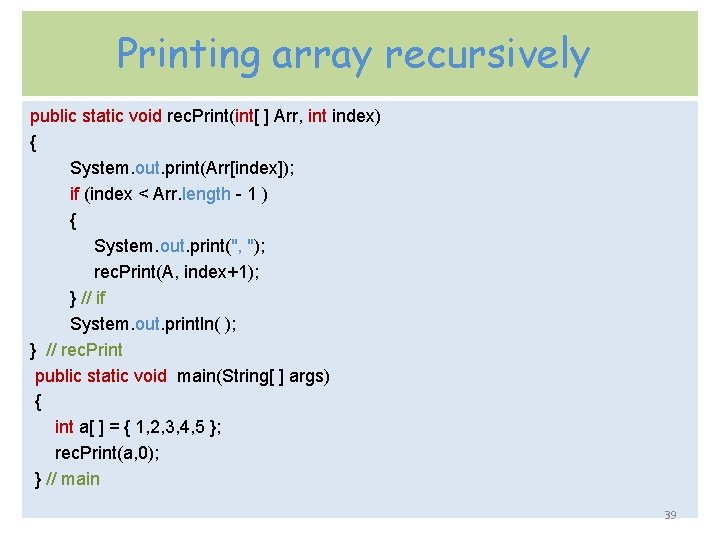Printing array recursively public static void rec. Print(int[ ] Arr, int index) { System. out. print(Arr[index]); if (index < Arr. length - 1 ) { System. out. print(", "); rec. Print(A, index+1); } // if System. out. println( ); } // rec. Print public static void main(String[ ] args) { int a[ ] = { 1, 2, 3, 4, 5 }; rec. Print(a, 0); } // main 39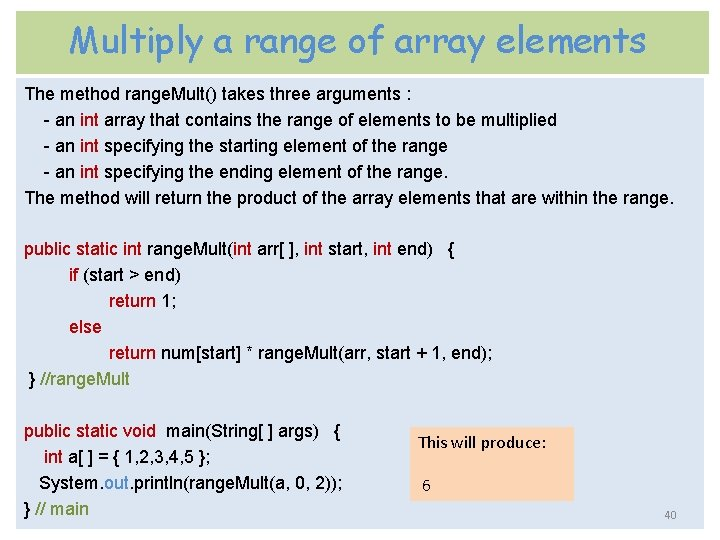Multiply a range of array elements The method range. Mult() takes three arguments : - an int array that contains the range of elements to be multiplied - an int specifying the starting element of the range - an int specifying the ending element of the range. The method will return the product of the array elements that are within the range. public static int range. Mult(int arr[ ], int start, int end) { if (start > end) return 1; else return num[start] * range. Mult(arr, start + 1, end); } //range. Mult public static void main(String[ ] args) { int a[ ] = { 1, 2, 3, 4, 5 }; System. out. println(range. Mult(a, 0, 2)); } // main This will produce: 6 40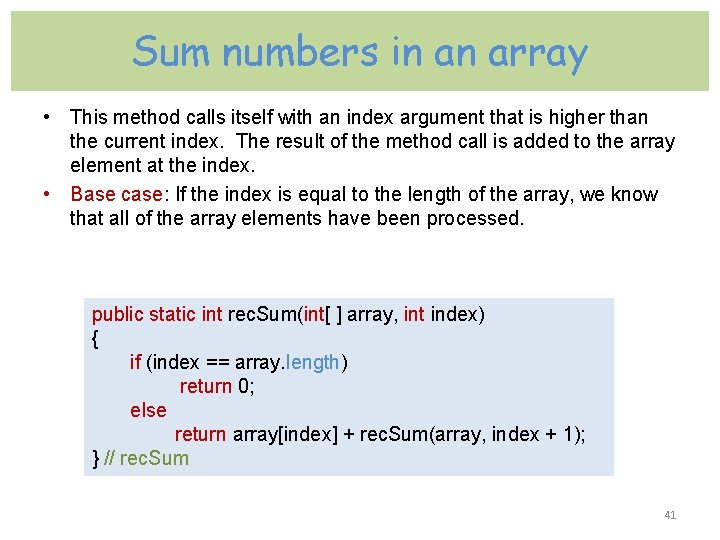Sum numbers in an array • This method calls itself with an index argument that is higher than the current index. The result of the method call is added to the array element at the index. • Base case: If the index is equal to the length of the array, we know that all of the array elements have been processed. public static int rec. Sum(int[ ] array, int index) { if (index == array. length) return 0; else return array[index] + rec. Sum(array, index + 1); } // rec. Sum 41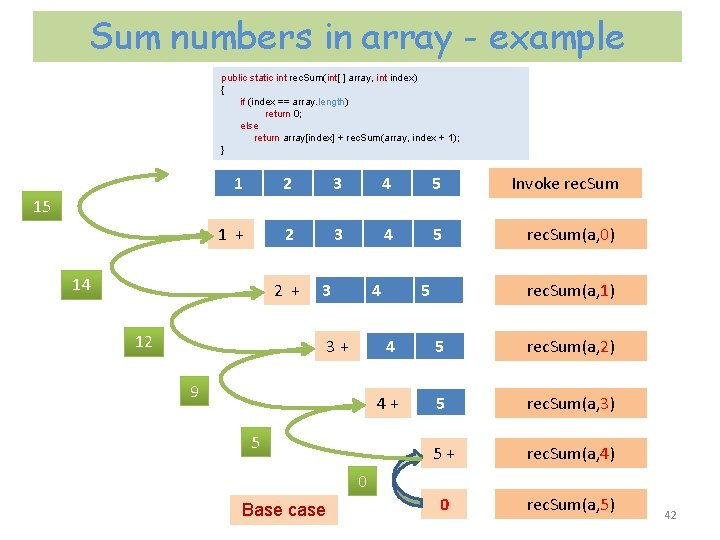Sum numbers in array - example public static int rec. Sum(int[ ] array, int index) { if (index == array. length) return 0; else return array[index] + rec. Sum(array, index + 1); } 1 2 3 4 5 Invoke rec. Sum 1 + 2 3 4 5 rec. Sum(a, 0) 15 14 2 + 3 12 4 3 + 9 5 5 rec. Sum(a, 1) 4 5 rec. Sum(a, 2) 4 + 5 rec. Sum(a, 3) 5 + rec. Sum(a, 4) 0 rec. Sum(a, 5) 0 Base case 42Find max value in an array The method gets an array of int value and it's length. It will return the max value in the array. public static int find. Max(int array[ ], int length) { if (length == 1) return array; // base case int result = find. Max(array, length - 1); if (array[length - 1] > result) return array[length - 1]; else return result; } // find. Max 43Find max value in an array - example public static int find. Max(int array[ ], int length) { if (length == 1) return array; // base case int result = find. Max(array, length - 1); if (array[length - 1] > result) return array[length - 1]; else return result; } index 0 1 2 value 2 7 5 public static int find. Max(array, 3) { if (3 == 1) F return array; // base case int result = find. Max(array, 2); if (array > result) return array; else public static int find. Max(array, 2) return result; { } if (2 == 1) F return array; // base case int result = find. Max(array, 1); if (array > result) return array; else return result; public static int find. Max(array, 1) } { if (1 == 1) T return array; // base case int result = find. Max(array, 0); if (array > result) return array; else return result; } arr Length=3 7 7 2 44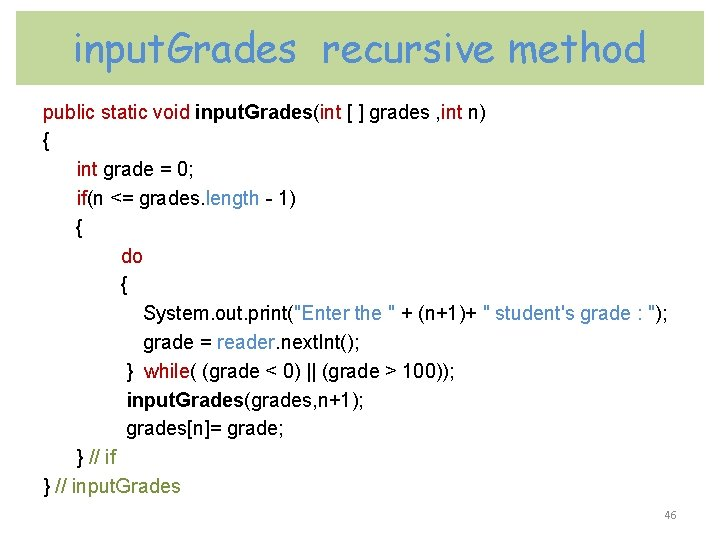print. Grades recursive method public static void print. Grades(int[ ] d, int m) { System. out. println( “The“+(m+1)+”student’s grade is " + d[m] ); if(m < d. length - 1) print. Grades(d, m+1); } //print. Grades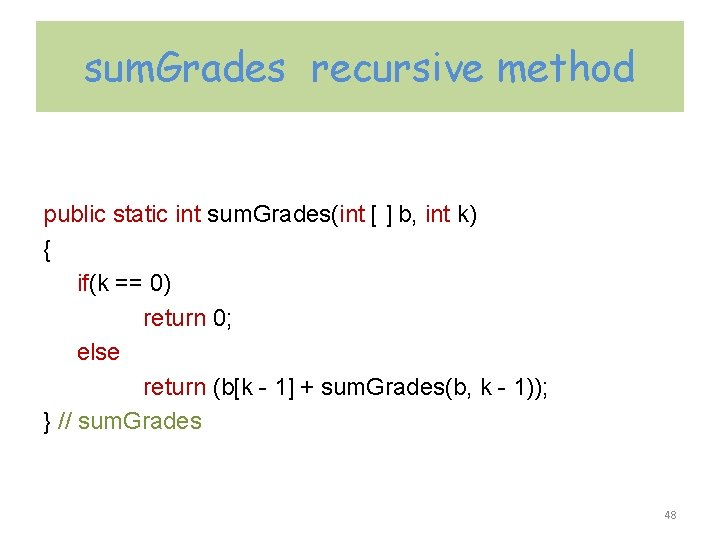sum. Grades recursive method public static int sum. Grades(int [ ] b, int k) { if(k == 0) return 0; else return (b[k - 1] + sum. Grades(b, k - 1)); } // sum. Grades 48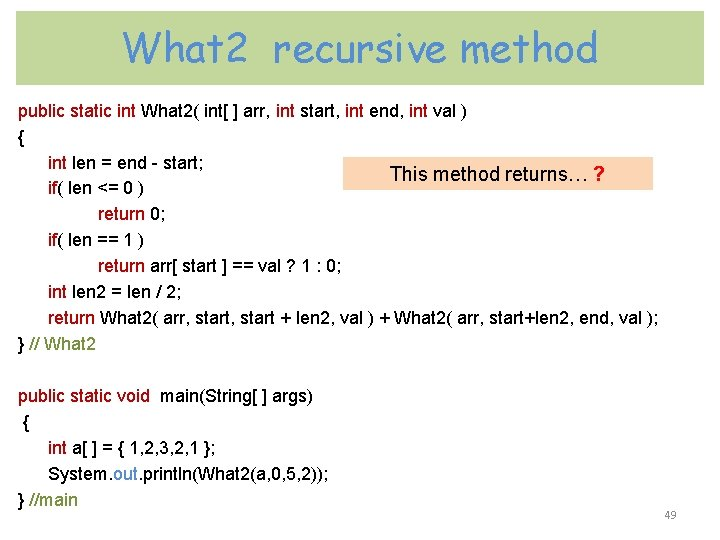What 2 recursive method public static int What 2( int[ ] arr, int start, int end, int val ) { int len = end - start; This method returns… ? if( len <= 0 ) return 0; if( len == 1 ) return arr[ start ] == val ? 1 : 0; int len 2 = len / 2; return What 2( arr, start + len 2, val ) + What 2( arr, start+len 2, end, val ); } // What 2 public static void main(String[ ] args) { int a[ ] = { 1, 2, 3, 2, 1 }; System. out. println(What 2(a, 0, 5, 2)); } //main 49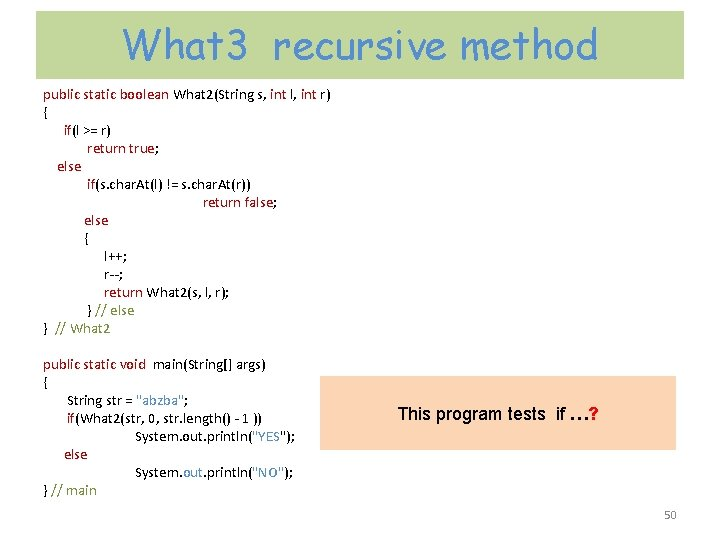What 3 recursive method public static boolean What 2(String s, int l, int r) { if(l >= r) return true; else if(s. char. At(l) != s. char. At(r)) return false; else { l++; r--; return What 2(s, l, r); } // else } // What 2 public static void main(String[] args) { String str = "abzba"; if(What 2(str, 0, str. length() - 1 )) System. out. println("YES"); else System. out. println("NO"); } // main This program tests if …? 50Recursive binary search This method returns the index of the entry if the target value is found or -1 if it is not found. public static int bin. Search( int a[ ], int target, int first, int last ) { int location = -1; //target not found; if(first <= last) { int mid=(first + last)/2; if(target == a[mid]) location = mid; else if(target < a[mid]) //first half location = bin. Search( a, target, first, mid - 1) else //second half location = bian. Search(a, target, mid + 1, last ) } return location; } //bin. Search 51Binary Search Example target is 33 The array a looks like this: Indexes 0 1 2 3 4 5 6 7 8 9 Contents 5 7 9 13 32 33 42 54 56 88 mid = (0 + 9) / 2 (which is 4) 33 > a[mid] (that is, 33 > a) So, if 33 is in the array, then 33 is one of: 5 6 7 8 9 33 42 54 56 88 Eliminated half of the remaining elements from consideration because array elements are sorted. 52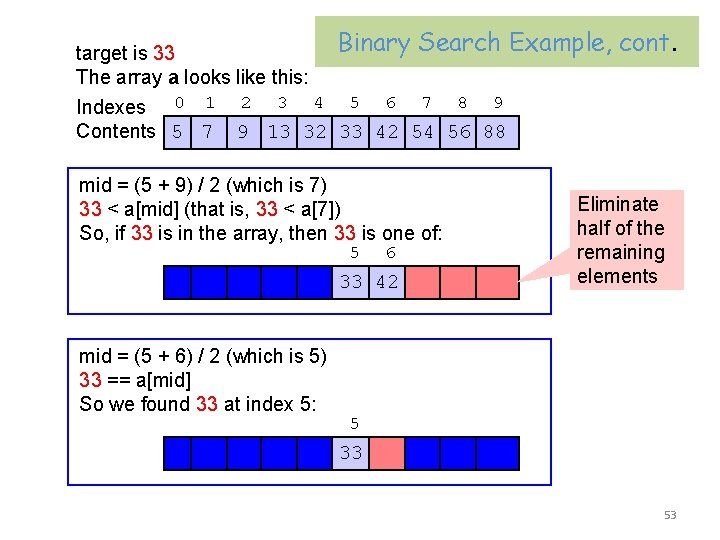Binary Search Example, target is 33 The array a looks like this: Indexes 0 1 2 3 4 5 6 7 8 9 Contents 5 7 9 13 32 33 42 54 56 88 mid = (5 + 9) / 2 (which is 7) 33 < a[mid] (that is, 33 < a) So, if 33 is in the array, then 33 is one of: 5 6 33 42 mid = (5 + 6) / 2 (which is 5) 33 == a[mid] So we found 33 at index 5: cont. Eliminate half of the remaining elements 5 33 53Bubble Sort - example 54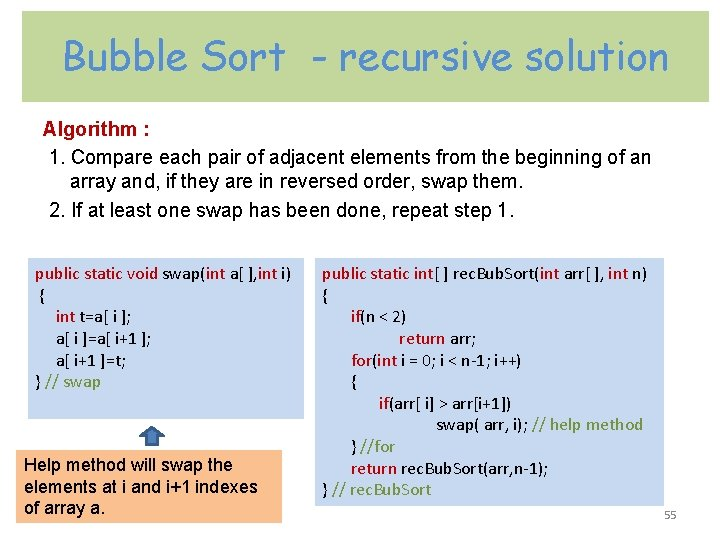Bubble Sort - recursive solution Algorithm : 1. Compare each pair of adjacent elements from the beginning of an array and, if they are in reversed order, swap them. 2. If at least one swap has been done, repeat step 1. public static void swap(int a[ ], int i) { int t=a[ i ]; a[ i ]=a[ i+1 ]; a[ i+1 ]=t; } // swap Help method will swap the elements at i and i+1 indexes of array a. public static int[ ] rec. Bub. Sort(int arr[ ], int n) { if(n < 2) return arr; for(int i = 0; i < n-1; i++) { if(arr[ i] > arr[i+1]) swap( arr, i); // help method } //for return rec. Bub. Sort(arr, n-1); } // rec. Bub. Sort 55Towers of Hanoi The Towers of Hanoi is a mathematical game or puzzle. It was invented by the French mathematician, Eduard Lucas, in 1883. 56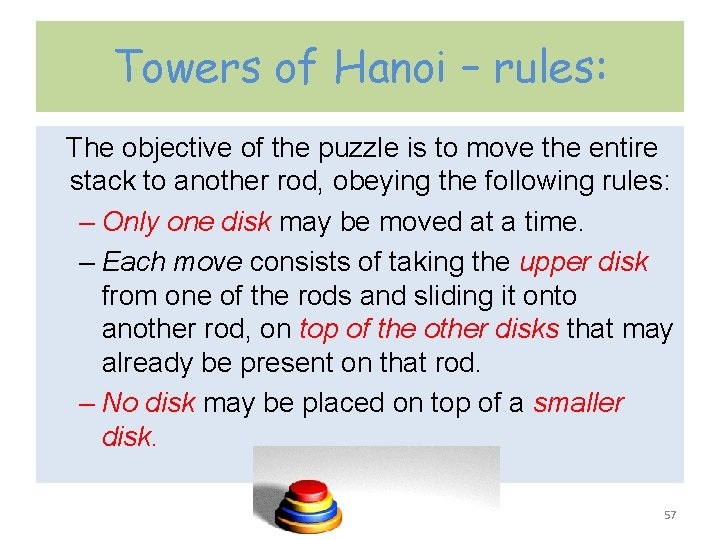Towers of Hanoi – rules: The objective of the puzzle is to move the entire stack to another rod, obeying the following rules: – Only one disk may be moved at a time. – Each move consists of taking the upper disk from one of the rods and sliding it onto another rod, on top of the other disks that may already be present on that rod. – No disk may be placed on top of a smaller disk. 57Recursive Algorithm To move n disks from peg a to peg c: move n− 1 disks from a to b. This leaves disk #n alone on peg a move disk #n from a to c move n− 1 disks from b to c so they sit on disk #n 58Towers of Hanoi – Java solution public static void hanoi (int x, char from, char to, char aux ) { if (x==1) System. out. println( "Move disk from “ + from + " to “ + to); else { hanoi(x-1, from, aux, to); System. out. println( "Move disk from “ + from+ " to “ + to); hanoi(x-1, aux, to, from); } } // hanoiTowers of Hanoi num=3 System. out. print( “Enter an integer “ ); int num = reader. next. Int(); hanoi(num, 'a' , 'c ' , 'b' ); 61M. C. Escher & Recursion Maurits Cornelis Escher ( 17 June 1898 – 27 March 1972) was a Dutch graphic artist. He is known for his often mathematically inspired , lithographs. “Drawing Hands” lithograph - illustrates the concept of Recursion. 62